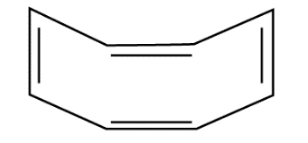# Assertion (A): The compound cyclooctane has the following structural`
Question:

Assertion (A): The compound cyclooctane has the following structural

formula:It is cyclic and has conjugated 8π-electron system but it is not

an aromatic compound.

Reason (R) : (4n + 2) π electrons rule does not hold good and the ring is not

planar.

(i) Both A and R are correct and R is the correct explanation of A.

(ii) Both A and R are correct but R is not the correct explanation of A.

(iii) Both A and R are not correct.

(iv) A is not correct but R is correct.

Solution:

Option (i) is correct.